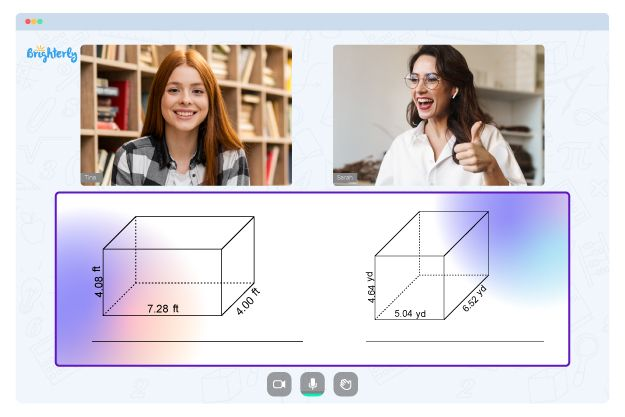# 45-45-90 And 30-60-90 Triangles Worksheets

Students who study our 45-45-90 and 30-60-90 triangles worksheets will learn about special right triangles and be able to solve related problems.

## What is 45-45-90 triangle?

A 45-45-90 triangle is a right triangle with  angles that are 45 degrees, 45 degrees, and 90 degrees. The ratio of the sides in a 45-45-90 triangle is always 1:1:sqrt(2). This means that if the length of one side of the triangle is “x,” then the length of the other two sides will also be “x.”

## What is 30-60-90 triangle?

Meanwhile, a 30-60-90 triangle is a right triangle with angles that are 30 degrees, 60 degrees, and 90 degrees. The ratio of the sides in a 30-60-90 triangle is always 1:sqrt(3):2. This means that if the length of the shorter leg (the side that is opposite the 30 degree angle) is “x,” then the length of the longer leg (the side that is opposite the 90 degree angle) is “x*sqrt(3)” and the hypotenuse is “2x”.

Math for Kids

Is Your Child Struggling With Math?
1:1 Online Math TutoringBoth of these triangles are special because of their unique side-angle relationships. This make them useful in solving geometric problems and in applied math like trigonometry. You can learn more from our 30-60-90 and 45-45-90 triangle worksheet.### 45-45-90 And 30-60-90 Triangles Worksheets PDF

45-45-90 And 30-60-90 Triangles Worksheet Answer Key### 45-45-90 And 30-60-90 Triangles Worksheets PDF

45-45-90 And 30-60-90 Triangle Worksheet### 45-45-90 And 30-60-90 Triangles Worksheets PDF

30 60 90 And 45 45 90 Triangle Worksheet With Answers### 45-45-90 And 30-60-90 Triangles Worksheets PDF

30-60-90 And 45-45-90 Triangle Worksheet

Our 45-45-90 and 30-60-90 triangle worksheet is free to download and is designed to make the concept of special right triangles easy for your kid to understand. You can also download our 30 60 90 and 45 45 90 triangle worksheet with answers. The 45-45-90 and 30-60-90 triangles worksheet answer key will help you kids to solve problems related to special right triangles.

Troubles with Geometry?• Does your child struggle to grasp geometry basics?
• Try learning with an online tutor.

Is your child having difficulties with understanding geometry lessons? An online tutor could provide the necessary help.